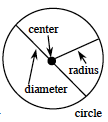### Home > CC2MN > Chapter 10 > Lesson 10.2.1 > Problem10-28

10-28.

Find the circumference of each of the circles described below.

1. Radius of $3$ inches

Recall that the circumference of a $\text{circle}=2\pi\left(\text{radius}\right)$.$2\pi\left(3\ \text{inches}\right)=6\pi\approx18.84$ inches

1. Diameter of $27$ cm

Refer to the diagram from part (a) and recall that the $\text{circumference}=\pi\left(\text{diameter}\right)$.

$27\pi$
To get an appropriate decimal answer, round $\pi$ so that it equals $3.14$.